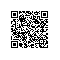# 深度学习利器：TensorFlow在智能终端中的应用——智能边缘计算，云端生成模型给移动端下载，然后用该模型进行预测

## 看花识名APPDaisy：雏菊

(点击放大图像)Dandelion：蒲公英

(点击放大图像)Roses：玫瑰

(点击放大图像)Sunflowers：向日葵

(点击放大图像)Tulips：郁金香

(点击放大图像)(点击放大图像)TensorFlow模型如何应用于看花识名APP中，主要包括以下几个关键步骤：模型选择和应用、模型文件转换以及Android开发。如下图所示：

(点击放大图像)(点击放大图像)## 模型训练及模型文件

(点击放大图像)• 首先下载Flowers数据，并转换为TFRecord格式：
DATA_DIR=/tmp/data/flowers
--dataset_dir="${DATA_DIR}" • 执行模型训练，经过36618次迭代后，模型精度达到85% TRAIN_DIR=/tmp/data/train python train_image_classifier.py --train_dir=${TRAIN_DIR}
--dataset_dir=${DATASET_DIR} --dataset_name=flowers --dataset_split_name=train --model_name=alexnet_v2 --preprocessing_name=vgg • 生成Inference Graph的PB文件 python export_inference_graph.py --alsologtostderr --model_name=alexnet_v2 --dataset_name=flowers --dataset_dir=${DATASET_DIR}
--output_file=alexnet_v2_inf_graph.pb
• 结合CheckPoint文件和Inference GraphPB文件，生成Freeze Graph的PB文件
python freeze_graph.py  --input_graph=alexnet_v2_inf_graph.pb
--input_checkpoint= \${TRAIN_DIR}/model.ckpt-36618  --input_binary=true
--output_graph=frozen_alexnet_v2.pb --output_node_names=alexnet_v2/fc8/squeezed
• 对Freeze Graph的PB文件进行数据量化处理，减少模型文件的大小，生成的quantized_alexnet_v2_graph.pb为智能终端中应用的模型文件
bazel-bin/tensorflow/tools/graph_transforms/transform_graph
--in_graph=frozen_alexnet_v2.pb  --outputs="alexnet_v2/fc8/squeezed"
strip_unused_nodes(type=float, shape="1,224,224,3")  remove_nodes(op=Identity,
op=CheckNumerics) fold_constants(ignore_errors=true)  fold_batch_norms
fold_old_batch_norms quantize_weights quantize_nodes
strip_unused_nodes sort_by_execution_order'

(点击放大图像)(点击放大图像)(点击放大图像)## TensorFlow Android应用开发环境构建

• 下载TensorFlow源代码

git clone --recurse-submodules https://github.com/tensorflow/tensorflow.git

• 下载安装Android NDK
• 下载安装Android SDK
• 配置tensorflow/WORKSPACE中android开发工具路径
android_sdk_repository(name = "androidsdk", api_level = 23, build_tools_version = "25.0.2", path = "/opt/android",)
android_ndk_repository(name="androidndk",  path="/opt/android/android-ndk-r12b",  api_level=14)
• 编译libtensorflow_inference.so
bazel build -c opt //tensorflow/contrib/android:libtensorflow_inference.so
--crosstool_top=//external:android/crosstool --host_crosstool_top=
@bazel_tools//tools/cpp:toolchain --cpu=armeabi-v7a
• 编译libandroid_tensorflow_inference_java.jar
bazel build //tensorflow/contrib/android:android_tensorflow_inference_java

TensorFlow提供了Android开发的示例框架，下面基于AlexNet模型的看花识名APP做一些相应源码的修改，并编译生成Android的安装包：

• 基于AlexNet模型，修改Inference的输入、输出的Tensor名称
private static final String INPUT_NAME = "input";
private static final String OUTPUT_NAME = "alexnet_v2/fc8/squeezed";
• 放置quantized_alexnet_v2_graph.pb和对应的labels.txt文件到assets目录下，并修改Android文件路径
private static final String MODEL_FILE = "file:///android_asset/quantized_alexnet_v2_graph.pb";
private static final String LABEL_FILE = "file:///android_asset/labels.txt";
• 编译生成安装包
bazel build -c opt //tensorflow/examples/android:tensorflow_demo
• 拷贝tensorflow_demo.apk到手机上，并执行安装，太阳花识别效果如下图所示：

(点击放大图像)## TensorFlow移动端应用开发API

• 构建TensorFlow Inference对象，构建该对象时候会加载TensorFlow动态链接库libtensorflow_inference.so到系统中；参数assetManager为android asset管理器；参数modelFilename为TensorFlow模型文件在android_asset中的路径。
TensorFlowInferenceInterface inferenceInterface = new
TensorFlowInferenceInterface(assetManager, modelFilename);
• 向TensorFlow图中加载输入数据，本App中输入数据为摄像头截取到的图片；参数inputName为TensorFlow Inference中的输入数据Tensor的名称；参数floatValues为输入图片的像素数据，进行预处理后的浮点值；[1,inputSize,inputSize,3]为裁剪后图片的大小，比如1张224*224*3的RGB图片。
inferenceInterface.feed(inputName, floatValues, 1, inputSize, inputSize, 3);
• 执行模型推理； outputNames为TensorFlow Inference模型中要运算Tensor的名称，本APP中为分类的Logist值。
inferenceInterface.run(outputNames);
• 获取模型Inference的运算结果，其中outputName为Tensor名称，参数outputs存储Tensor的运算结果。本APP中，outputs为计算得到的Logist浮点数组。
inferenceInterface.fetch(outputName, outputs);

## 参考文献使用钉钉扫一扫加入圈子
+ 订阅